## 3-D Solution and Gradient Plots with MATLAB® Functions

### Types of 3-D Solution Plots Available in MATLAB

In addition to surface and gradient plots available with the PDE plotting functions, you can use MATLAB® graphics capabilities to create more types of plots for your 3-D model.

### 2-D Slices Through 3-D Geometry

This example shows how to obtain plots from 2-D slices through a 3-D geometry.

The problem is

`$\frac{\partial u}{\partial t}-\Delta u=f$`

on a 3-D slab with dimensions 10-by-10-by-1, where $u=0$ at time `t = 0`, boundary conditions are Dirichlet, and

`$f\left(x,y,z\right)=1+y+10{z}^{2}$`

Set Up and Solve the PDE

Define a function for the nonlinear `f` coefficient in the syntax as given in f Coefficient for specifyCoefficients.

`function bcMatrix = myfffun(region,state)`

`bcMatrix = 1+10*region.z.^2+region.y;`

Import the geometry and examine the face labels.

```model = createpde; g = importGeometry(model,'Plate10x10x1.stl'); pdegplot(g,'FaceLabels','on','FaceAlpha',0.5)```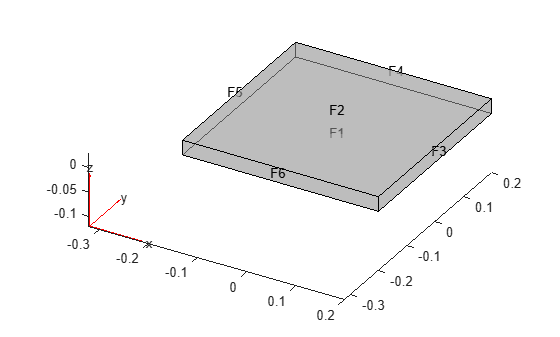The faces are numbered 1 through 6.

Create the coefficients and boundary conditions.

```c = 1; a = 0; d = 1; f = @myfffun; specifyCoefficients(model,'m',0,'d',d,'c',c,'a',a,'f',f); applyBoundaryCondition(model,'dirichlet','face',1:6,'u',0);```

Set a zero initial condition.

`setInitialConditions(model,0);`

Create a mesh with sides no longer than 0.3.

`generateMesh(model,'Hmax',0.3);`

Set times from 0 through 0.2 and solve the PDE.

```tlist = 0:0.02:0.2; results = solvepde(model,tlist);```

Plot Slices Through the Solution

Create a grid of `(x,y,z)` points, where `x = 5`, `y` ranges from 0 through 10, and `z` ranges from 0 through 1. Interpolate the solution to these grid points and all times.

```yy = 0:0.5:10; zz = 0:0.25:1; [YY,ZZ] = meshgrid(yy,zz); XX = 5*ones(size(YY)); uintrp = interpolateSolution(results,XX,YY,ZZ,1:length(tlist));```

The solution matrix `uintrp` has 11 columns, one for each time in `tlist`. Take the interpolated solution for the second column, which corresponds to time 0.02.

`usol = uintrp(:,2);`

The elements of `usol` come from interpolating the solution to the `XX`, `YY`, and `ZZ` matrices, which are each 5-by-21, corresponding to `z-by-y` variables. Reshape `usol` to the same 5-by-21 size, and make a surface plot of the solution. Also make surface plots corresponding to times 0.06, 0.10, and 0.20.

```figure usol = reshape(usol,size(XX)); subplot(2,2,1) surf(usol) title('t = 0.02') zlim([0,1.5]) xlim([1,21]) ylim([1,5]) usol = uintrp(:,4); usol = reshape(usol,size(XX)); subplot(2,2,2) surf(usol) title('t = 0.06') zlim([0,1.5]) xlim([1,21]) ylim([1,5]) usol = uintrp(:,6); usol = reshape(usol,size(XX)); subplot(2,2,3) surf(usol) title('t = 0.10') zlim([0,1.5]) xlim([1,21]) ylim([1,5]) usol = uintrp(:,11); usol = reshape(usol,size(XX)); subplot(2,2,4) surf(usol) title('t = 0.20') zlim([0,1.5]) xlim([1,21]) ylim([1,5])```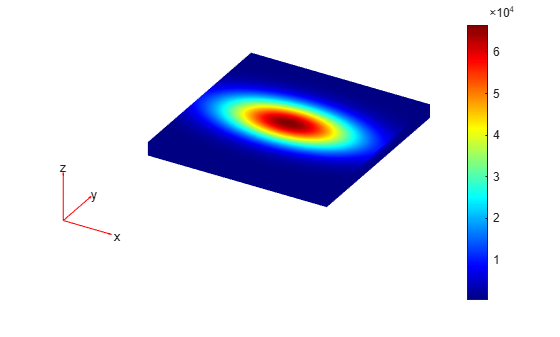### Contour Slices Through 3-D Solution

This example shows how to create contour slices in various directions through a solution in 3-D geometry.

Set Up and Solve the PDE

The problem is to solve Poisson's equation with zero Dirichlet boundary conditions for a complicated geometry. Poisson's equation is

`$-\nabla \cdot \nabla u=f.$`

Partial Differential Equation Toolbox™ solves equations in the form

`$-\nabla \cdot \nabla \left(cu\right)+au=f.$`

So you can represent the problem by setting $c=1$ and $a=0$. Arbitrarily set $f=10$.

```c = 1; a = 0; f = 10;```

The first step in solving any 3-D PDE problem is to create a PDE Model. This is a container that holds the number of equations, geometry, mesh, and boundary conditions for your PDE. Create the model, then import the `'ForearmLink.stl'` file and view the geometry.

```N = 1; model = createpde(N); importGeometry(model,'ForearmLink.stl'); pdegplot(model,'FaceAlpha',0.5) view(-42,24)```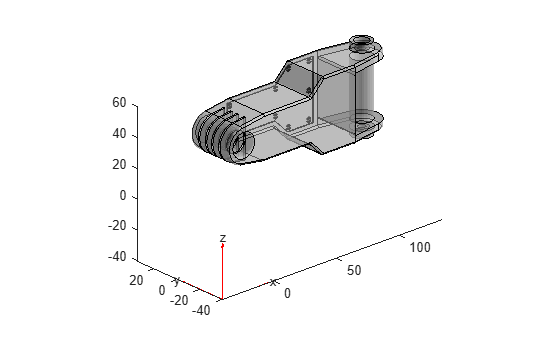Specify PDE Coefficients

Include the PDE coefficients in `model`.

`specifyCoefficients(model,'m',0,'d',0,'c',c,'a',a,'f',f);`

Create zero Dirichlet boundary conditions on all faces.

`applyBoundaryCondition(model,'dirichlet','Face',1:model.Geometry.NumFaces,'u',0);`

Create a mesh and solve the PDE.

```generateMesh(model); result = solvepde(model);```

Plot the Solution as Contour Slices

Because the boundary conditions are $u=0$ on all faces, the solution $u$ is nonzero only in the interior. To examine the interior, take a rectangular grid that covers the geometry with a spacing of one unit in each coordinate direction.

`[X,Y,Z] = meshgrid(0:135,0:35,0:61);`

For plotting and analysis, create a `PDEResults` object from the solution. Interpolate the result at every grid point.

```V = interpolateSolution(result,X,Y,Z); V = reshape(V,size(X));```

Plot contour slices for various values of $z$.

```figure colormap jet contourslice(X,Y,Z,V,[],[],0:5:60) xlabel('x') ylabel('y') zlabel('z') colorbar view(-11,14) axis equal```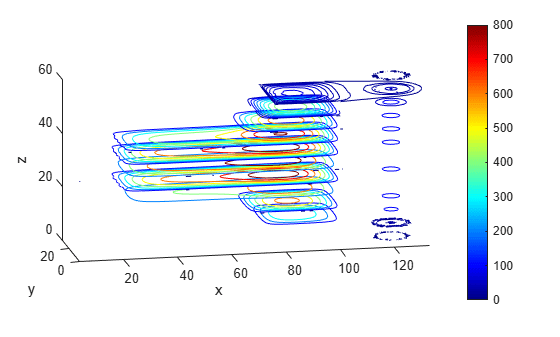Plot contour slices for various values of $y$.

```figure colormap jet contourslice(X,Y,Z,V,[],1:6:31,[]) xlabel('x') ylabel('y') zlabel('z') colorbar view(-62,34) axis equal```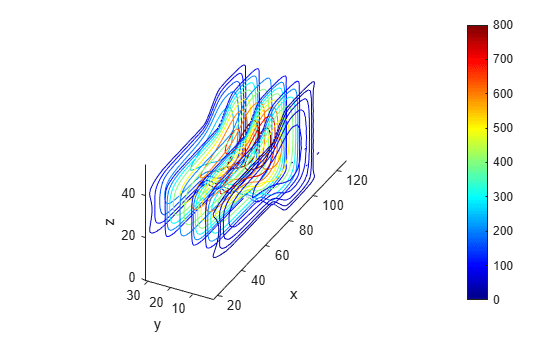Save Memory by Evaluating As Needed

For large problems you can run out of memory when creating a fine 3-D grid. Furthermore, it can be time-consuming to evaluate the solution on a full grid. To save memory and time, evaluate only at the points you plot. You can also use this technique to interpolate to tilted grids, or to other surfaces.

For example, interpolate the solution to a grid on the tilted plane $0\le x\le 135$, $0\le y\le 35$, and $z=x/10+y/2$. Plot both contours and colored surface data. Use a fine grid, with spacing 0.2.

```[X,Y] = meshgrid(0:0.2:135,0:0.2:35); Z = X/10 + Y/2; V = interpolateSolution(result,X,Y,Z); V = reshape(V,size(X)); figure subplot(2,1,1) contour(X,Y,V); axis equal title('Contour Plot on Tilted Plane') xlabel('x') ylabel('y') colorbar subplot(2,1,2) surf(X,Y,V,'LineStyle','none'); axis equal view(0,90) title('Colored Plot on Tilted Plane') xlabel('x') ylabel('y') colorbar```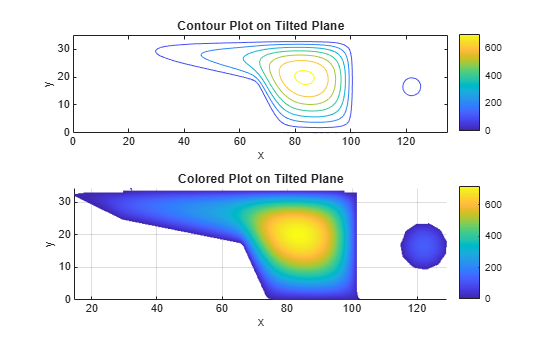### Plots of Gradients and Streamlines

This example shows how to calculate the approximate gradients of a solution, and how to use those gradients in a quiver plot or streamline plot.

The problem is the calculation of the mean exit time of a Brownian particle from a region that contains absorbing (escape) boundaries and reflecting boundaries. For more information, see Narrow escape problem. The PDE is Poisson's equation with constant coefficients. The geometry is a simple rectangular solid. The solution $u\left(x,y,z\right)$ represents the mean time it takes a particle starting at position $\left(x,y,z\right)$ to exit the region.

Import and View the Geometry

```model = createpde; importGeometry(model,'Block.stl'); pdegplot(model,'FaceLabels','on','FaceAlpha',0.5) view(-42,24)```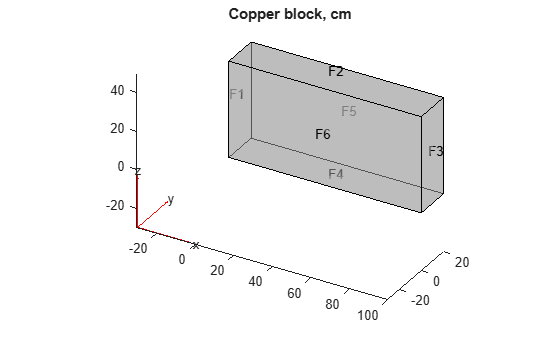Set Boundary Conditions

Set faces 1, 2, and 5 to be the places where the particle can escape. On these faces, the solution $u=0$. Keep the default reflecting boundary conditions on faces 3, 4, and 6.

`applyBoundaryCondition(model,'dirichlet','Face',[1,2,5],'u',0);`

Create PDE Coefficients

The PDE is

`$-\Delta u=-\nabla \cdot \nabla u=2$`

In Partial Differential Equation Toolbox™ syntax,

`$-\nabla \cdot \left(c\nabla u\right)+au=f$`

This equation translates to coefficients `c = 1`, `a = 0`, and `f = 2`. Enter the coefficients.

```c = 1; a = 0; f = 2; specifyCoefficients(model,'m',0,'d',0,'c',c','a',a,'f',f);```

Create Mesh and Solve PDE

Initialize the mesh.

`generateMesh(model);`

Solve the PDE.

`results = solvepde(model);`

Examine the Solution in a Contour Slice Plot

Create a grid and interpolate the solution to the grid.

```[X,Y,Z] = meshgrid(0:135,0:35,0:61); V = interpolateSolution(results,X,Y,Z); V = reshape(V,size(X));```

Create a contour slice plot for five fixed values of the `y`-coordinate.

```figure colormap jet contourslice(X,Y,Z,V,[],0:4:16,[]) xlabel('x') ylabel('y') zlabel('z') xlim([0,100]) ylim([0,20]) zlim([0,50]) axis equal view(-50,22) colorbar```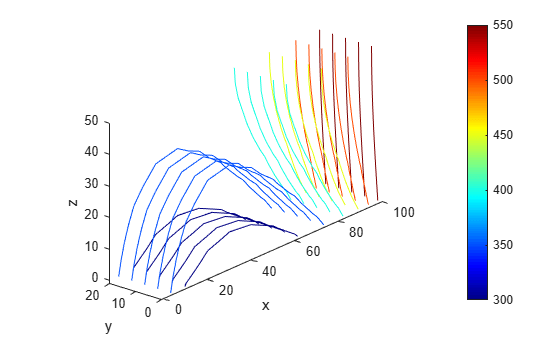The particle has the largest mean exit time near the point $\left(x,y,z\right)=\left(100,0,0\right)$.

Use Gradients for Quiver and Streamline Plots

Examine the solution in more detail by evaluating the gradient of the solution. Use a rather coarse mesh so that you can see the details on the quiver and streamline plots.

```[X,Y,Z] = meshgrid(1:9:99,1:3:20,1:6:50); [gradx,grady,gradz] = evaluateGradient(results,X,Y,Z);```

Plot the gradient vectors. First reshape the approximate gradients to the shape of the mesh.

```gradx = reshape(gradx,size(X)); grady = reshape(grady,size(Y)); gradz = reshape(gradz,size(Z)); figure quiver3(X,Y,Z,gradx,grady,gradz) axis equal xlabel 'x' ylabel 'y' zlabel 'z' title('Quiver Plot of Estimated Gradient of Solution')```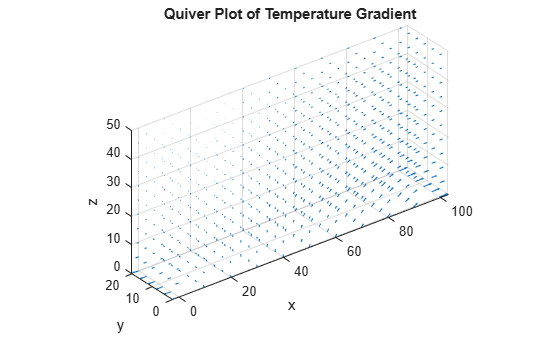Plot the streamlines of the approximate gradient. Start the streamlines from a sparser set of initial points.

```hold on [sx,sy,sz] = meshgrid([1,46],1:6:20,1:12:50); streamline(X,Y,Z,gradx,grady,gradz,sx,sy,sz) title('Quiver Plot with Streamlines') hold off```The streamlines show that small values of `y` and `z` give larger mean exit times. They also show that the `x`-coordinate has a significant effect on `u` when `x` is small, but when `x` is greater than 40, the larger values have little effect on `u`. Similarly, when `z` is less than 20, its values have little effect on `u`.

## Support